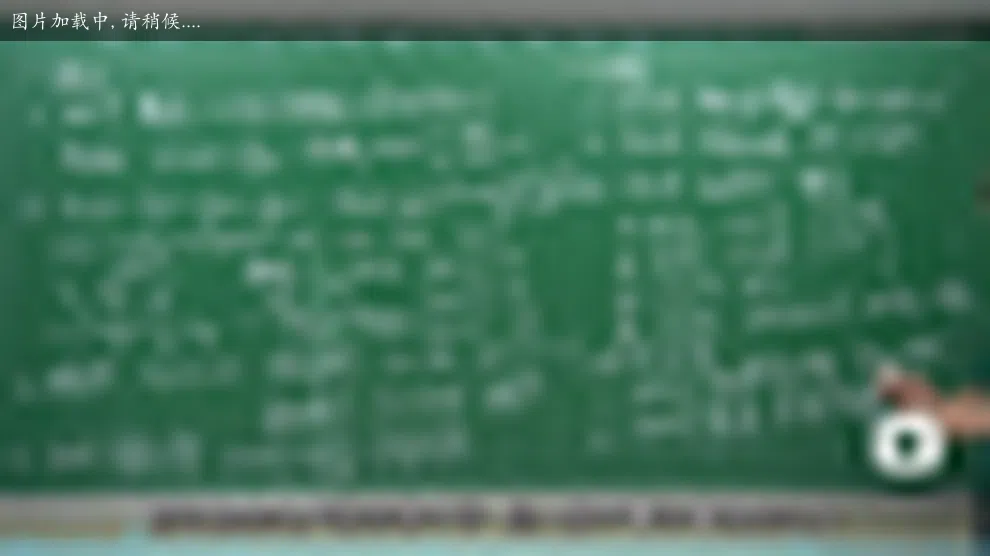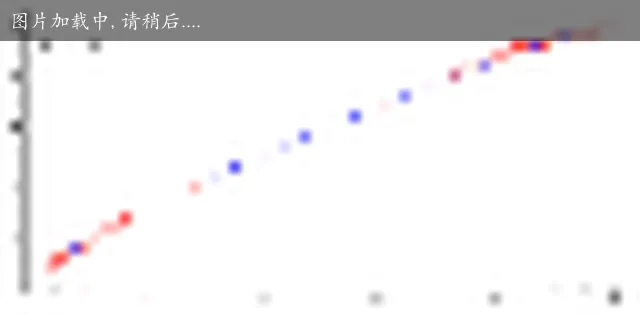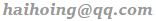$\zeta$ 函数 $$\zeta(s)=1+\frac{1}{2^s}+\frac{1}{3^s}+\frac{1}{4^s}+\cdots\\$$

• 当 $s=1$ 时，就变成了著名的调和级数，是不收敛的。
• 当 $s<1$ 时，加的项会逐渐变大，最后的结果会趋于正无穷，也是不收敛的。
• 当 $s>1$ 时，加的项越来越小，可以想象它最后会趋于一个实数，我们称这种趋于一个数的情况为收敛的。$J(x)=Li(x)-\sum_{\rho}Li(x^{\rho})-\ln 2 +\int_x^\infty\frac{dt}{t(t^2-1)\ln t}$## 李永乐老师

1. 需要处处可导
• 给数学系用的复变函数教程上都会讲到，只要在一个有聚点的点集上给定了函数值，那么无论怎样做解析延拓，都只能有唯一的结果。
• 任何两个全纯函数，如果在一个区域内相等，则它们在任何一点处都相等。因此，解析延拓是唯一的。

## 3Blue1Brown

【官方双语】黎曼 ζ 函数与解析延拓的可视化## 实际应用

If you have any questions or feedback, please reach out.